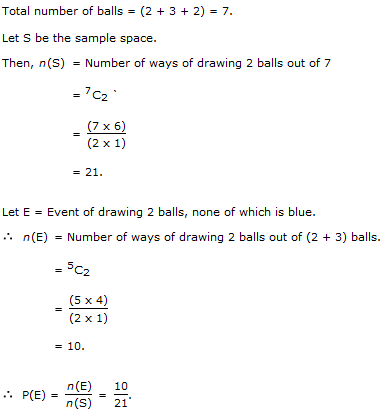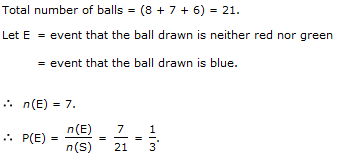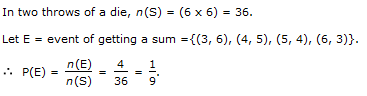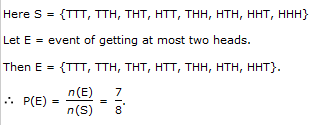1.

Tickets numbered 1 to 20 are mixed up and then a ticket is drawn at random. What is the probability that the ticket drawn has a number which is a multiple of 3 or 5?

 A. 1/2 B. 2/5 C. 8/15 D. 9/20
Explanation: Here, S = {1, 2, 3, 4, ...., 19, 20}.

Let E = event of getting a multiple of 3 or 5 = {3, 6 , 9, 12, 15, 18, 5, 10, 20}.

P(E) = n(E)/n(S) = 9/20.

2.

A bag contains 2 red, 3 green and 2 blue balls. Two balls are drawn at random. What is the probability that none of the balls drawn is blue?

 A. 10/21 B. 11/21 C. 2/7 D. 5/7
Explanation:3.

In a box, there are 8 red, 7 blue and 6 green balls. One ball is picked up randomly. What is the probability that it is neither red nor green?

 A. 1/3 B. 3/4 C. 7/19 D. 8/21
Explanation:4.

What is the probability of getting a sum 9 from two throws of a dice?

 A. 1/6 B. 1/8 C. 1/9 D. 1/12
Explanation:Explanation: